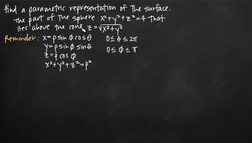PLAY PREVIEW

### Segments in this Video

#### Parametric Representation of the Sphere (00:50)

FREE PREVIEW

We need to find parametric equations that represent the part of the sphere that's above the cone. Since the surface of a sphere is two dimensional, parametric equations usually have two variables.

#### Converting Equations to Parameter Values(00:47)

We want to convert the equation of a sphere into parametric equations in terms of phi and theta, and solve for rho.

#### Finding the Value of Rho(01:03)

In spherical coordinates, rho represents the radius of the sphere. When dealing with three dimensional coordinate space, there can not be a negative radius so, the sphere will not exist.

#### Parametric Equations that Represent the Sphere(01:19)

When looking at the top part of the sphere, the interval in which theta and pi are defined need to be limited.

#### Horizontal Cross Section(01:03)

We can redefine the interval for phi so that it only includes positive values, in order to better match the equation for the cone.

#### Putting Everything in Terms of Pi to Redefine the Interval(02:35)

Everything needs to be in terms of Pi to redefine the interval for Pi. We will use the parametric equations then simplify. Then consult the unit circle to see where the answer is true.

#### Defining a Parametric Equation(00:50)

The parametric representation of a surface is a set of equations that define the surface in terms of two variables, theta and phi, which represent the location on the surface relative to some starting point.

#### Credits(00:00)

Credits

For additional digital leasing and purchase options contact a media consultant at 800-257-5126
(press option 3) or sales@films.com.

# Parametric representation of the surface

Part of the Series : Integral Calc: Calculus 3
 3-Year Streaming Price: \$49.95

Share

### Description

This video tutorial works through math problems/equations that address topics in Calculus 3, Vectors. This specific tutorial addresses Parametric representation of the surface.

Length: 9 minutes

Item#: BVL275740Closed Captioned